## 4(x+9)=2x-6 Solve for x

Question

4(x+9)=2x-6
Solve for x

in progress 0
5 months 2021-08-15T07:05:30+00:00 2 Answers 4 views 0

1. Hi there!

We are given the equation below: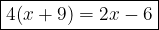1. Expand 4 in the expression.

• When expand in the expression, it is like multiply everything in the expression. So when we expand 4 in x+9, it becomes 4(x)+9(4).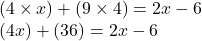Cancel the brackets.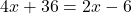2. Isolate xterm and solve for the variable.

• Think it easy. If you want to isolate x-term then what should you do? Well simply swap sides, and change the operator/sign.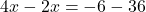Finally, combine like terms.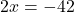Then divide both sides by 2 so we can finally leave only x-term.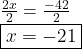3. Check the solution if it is right or wrong.

• This step is optional but if you are not confident on your answer, this step is recommended.

To check the answer, we simply substitute the value of x which is -21 in the equation and see if both sides are equal or not. If both sides are equal then the answer is correct, if not then the answer is wrong. Therefore,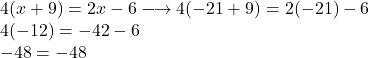Since both sides are equal when substitute in x = -21.

• Hence, the answer for this equation is x = 21.

I hope this helps and let me know if you have any doubts!

-21

Step-by-step explanation:

4(x+9) = 4x+36

4x+36 = 2x-6

-36        -36

minus 36 from both sides

4x = 2x-42

2x = -42

-42/2 = -21

x = -21